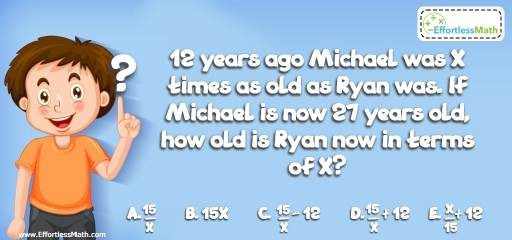# Algebra Puzzle – Challenge 48

This is an interactive mathematical and logic puzzle prepared for anyone interested in school-level math and critical thinking.## Challenge:

12 years ago Michael was X times as old as Ryan was. If Michael is now 27 years old, how old is Ryan now in terms of X?

A- $$\frac{x}{15}$$

B- $$15x$$

C- $$\frac{15}{x}-12$$

D- $$\frac{15}{x}+12$$

E- $$\frac{x}{15}+12$$

### The Absolute Best Book to challenge your Smart Student!

12 years ago Michael was 15 years old. At that time, he was X times as old as Ryan was. Plug is a value for X. Let’s say X is 3. Therefore, Ryan was 5 years old 12 years ago. Now, Ryan is 17.
Plug in 3 for X and check all 5 options:
A. $$\frac{15}{3} = 3$$,
B. $$15 × 3 = 45$$,
C. $$\frac{15}{3} – 12= -7$$,
D. $$\frac{15}{3} + 12 = 17$$! Bingo
E. $$\frac{3}{15} + 12 = \frac{123}{15}$$

### What people say about "Algebra Puzzle – Challenge 48 - Effortless Math: We Help Students Learn to LOVE Mathematics"?

No one replied yet.

X
30% OFF

Limited time only!

Save Over 30%

SAVE $5 It was$16.99 now it is \$11.99